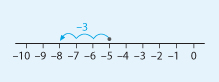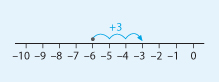Succeed with maths – Part 1

Start this free course now. Just create an account and sign in. Enrol and complete the course for a free statement of participation or digital badge if available.

Free course

# 5 Rules for negatives

As you first learn how to work with negative numbers and develop your skills, a number line is very helpful – but it can sometimes be very tedious and not very convenient!

So, remembering a few basic rules can be a great help.

Rule 1

If you are adding two numbers that have the same sign, like (–4) + (–3) , momentarily ignore the signs, and add the numbers together. Then place the sign in front of the sum.

You can think of (–4) + (–3) as 4 + 3, which equals 7. Since both numbers were negative, you place a negative sign in front of the answer: (–4) + (–3) = –7. You can verify this using a number line.

Rule 2

If you are adding two numbers that have different signs, such as (–8) + 2, you momentarily ignore the signs. Then subtract the numbers and place the sign of the ‘larger’ number in front of the difference.

You ignore the signs in (–8) + 2 and subtract 8 − 2, which equals 6. Since the ‘larger number’ is 8, and it has a negative sign in front of it, the overall answer is also negative: (–8) + 2 = –6.

Rule 3

If you are subtracting a positive number, like (–5) − 3, then rewrite it as addition of the negative number and then use the rules for addition: (–5) − 3 = (–5) + (–3) = –8.

To help you visualise this example, look at the number line in Figure 17.Figure _unit8.5.1 Figure 17 The sum (–5) – 3 = –8

Rule 4

If you are subtracting a negative number, such as (–6) − (–3), then rewrite it as addition of the positive number and then use the rules for addition: (–6) − (–3) = (–6) + 3 = –3.

Check out the number line in Figure 18 to see the addition.Figure _unit8.5.2 Figure 18 The sum (–6) − (–3) = –3

Of course, if you feel comfortable adding and subtracting negative without these rules, there is no need to use them.

Use the rules in this next activity to help you to decide if you find them useful.

## Activity _unit8.5.1 Activity 6 Adding and subtracting negative numbers using the four strategies

Timing: Allow approximately 10 minutes

Try these questions using the four strategies above.

• a.18 + (–3)

### Discussion

Look at Rule 2 above.

• a.You are adding two numbers with different signs. Ignore the signs and subtract the numbers  18 − 3 and use the sign from the larger number in the answer. So, 18 − 3 = 15.
• b.(–21) + (–6)

### Discussion

Look at Rule 1 above.

• b.You are adding two numbers with the same sign. Ignore the signs and add the numbers, 21 + 6, and put back the signs from the numbers. So, (–21) + (–6) = –27.
• c.32 − (–8)

### Discussion

Look at Rule 4.

• c.Rewrite as addition of a positive number, 32 + 8. So, 32 − (–8) = 40.
• d.(–25) − (–4)

• d.Rewrite as addition of a positive number, –25 + 4. Then follow rule 2. Ignore the signs and subtract the numbers 25 − 4, and use the sign from the larger number in the answer.

(–25) − (–4) = –21

It was important to get to grips with carrying out addition and subtraction involving negative numbers on paper or in your head to get a real understanding of how to do this. It will help if you keep the rules to hand and practise your new skills. Fortunately, you can also turn to a calculator. You can try this in the next section.